Encoding tables for constants

A constant is encoded as a series of instructions, where each instruction defines a digit of the constant, from the least significant to the most significant.

Each instruction has a right part defining the digit, and a left part defining its attributes.

Right part of the instruction

S

0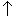1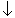2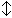3

+

4

-

5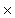6

:

7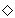8

*

9

Left part of the instruction
Last digit?  Sign Decimal point  Code

Yes

+
Yes  D/
No  D

-
Yes  E/
No  E

No

+
Yes  R/
No  R

-
Yes  F/
No

Examples of encoded constants:

250.34 must be encoded as:

 A  /Marks the start of an encoded constant R + 4 + says it's a four R says it's not the last digit, it's a positive number, and it doesn't have the decimal point R3says it's a three R says it's not the last digit, it's a positive number, and it doesn't have the decimal point R / S 0. S says it's a zero R / says it's not the last digit, it's a positive number, and has the decimal point (i.e., this is the digit that expresses units) R  - 5 - says it's a five R says it's not the last digit, it's a positive number, and it doesn't have the decimal point D2says it's a two D says it is the last digit, it's a positive number, and it doesn't have the decimal point

-10 must be encoded as:

 A  /Marks the start of an encoded constant F / S 0. S says it's a zero F / says it's not the last digit, it's a negative number, and has the decimal point (i.e., this is the digit that expresses units) E  - 1 - says it's a one E says it is the last digit, it's a negative number, and it doesn't have the decimal point

1 must be encoded as:

 A  /Marks the start of an encoded constant D /1.says it's a one D / says it is the last digit, it's a positive number, and has the decimal point (i.e., this is the digit that expresses units)

This page is part of the Programma 101 Web